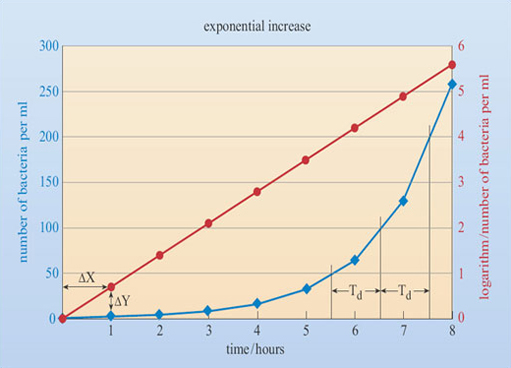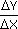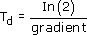Using numbers and handling data

Start this free course now. Just create an account and sign in. Enrol and complete the course for a free statement of participation or digital badge if available.

Free course

# 3.6.4 Representing exponential relationships using graphs

What do exponential increase and decrease look like when plotted as a graph? Although exponentials describe anything that continually doubles or halves, the specific assumption of 'exponential increase' and 'exponential decay' are that these happen during a constant time interval. If the time taken for doubling or halving remains constant, then an exponential increase looks like the thick blue line in Figure 12, which shows the number of bacteria present in a millilitre of growth medium (marked on the left side of the graph), counted each hour.Figure 12 Graph showing an exponential increase of bacteria numbers over time

You can see that at 3 hours there were about 10 bacteria/ml, at 4 hours there were about 20 bacteria/ml and at 5 hours there were about 40 bacteria/ml, so, in this case the bacteria are dividing with a doubling time of around 1 hour. In practice, it's unlikely that any cell type would be doubling, rather conveniently, at each time point you had chosen to make a measurement. Instead, it's best to estimate the doubling time by reading off the time at which there were a known number of cells e.g. 50 bacteria/ml, and then reading off the time when that number of bacteria had doubled to 100 bacteria/ml. The time difference between these values gives the doubling time (often given the symbol, Td) or if we were dealing with exponential decay, it would give the half-life (often given the symbol, t1/2). In this case, there were 50 bacteria/ml at about 5.5 hours and 100 bacteria/ml at about 6.5 hours, so the bacteria doubled in about 6.5 - 5.5 = 1 hour. Try this for yourself to estimate how long it took to go from 100 bacteria/ml to 200 bacteria/ml. If the bacteria are still growing exponentially then you should get the same value for the doubling time.

Because an exponential increase can also be represented as a power series, if we drew a graph showing the time at which there were 21, 22, 23, 24, 25, 26, 27, etc. bacteria present then this would produce a straight line if the bacteria were doubling exponentially. In practice, it's extremely unlikely that we would be lucky enough to make a measurement when there were exactly 4 (i.e. 22) or 16 (24) or 128 (27) bacteria present. More likely, we might find that we had 130 bacteria/ml instead of 128 bacteria/ml. Nevertheless, it is possible to convert our existing data of bacteria number measured at fixed time points into a straight-line graph. To do this we need to convert our bacteria number values into logarithms.

A logarithm isn't a lumberjack pop group, but is a mathematical method to calculate the values of exponents. In essence, a logarithm asks the question 'what power do I need to raise something to in order to get the answer I want?' For instance, 2 raised to what power, 'p', gives the value 128? (i.e. 128 = 2p). We've already seen that 128 = 27, so the logarithm of 128 with base 2 is 7.

A word about bases: you're already familiar with the decimal system of numbering, in which exponents are of the type 'ten to the power something' (e.g. 103, 106 etc.). In the decimal system, 10 is the base number. However, we could actually choose any base we like. Things that change by halving or doubling are best expressed using base 2, i.e. you'd express exponents in a base 2 system of numbering as 'two to the power something' (23, 26, etc.).

For many complex historical and mathematical reasons, most logarithms use a base value of 2.718, a special mathematical constant. For instance, if you use the logarithm button on a calculator (marked 'ln', or 'natural logarithm') to find the logarithm of 128 you are asking it to calculate the value of 'p' in the equation 128 = 2.718p. This calculation gives the answer p = 4.852.

In Figure 12 we have converted the measurements of the numbers of bacteria/ml into their logarithm values (marked on the right side of the graph) and have plotted these against time, using a red colour. Because one axis is logarithmic and the other is normal, this is called a semi-logarithmic graph. Notice that plotting the logarithmic data gives a straight line, as we predicted it would, and this confirms that the growth of the bacteria is exponential. If their growth had started to slow down then the semi-logarithmic plot would deviate from a straight line at that point and begin to flatten off. It is for this reason - that it's easier to tell by eye if a line is straight than to tell if it's an exponential curve - that semi-logarithmic graphs are used to spot this kind of pattern.

The steepness, or gradient, of the line in a semi-logarithmic graph can be used to determine how fast the bacteria are dividing, i.e. to find the doubling time, or in the case of exponential decay to find the half-life. You may come across specific names for this gradient: for exponential increases, the gradient is also known as the growth constant, whilst for exponential decreases the gradient is known as the decay constant.

Just like the gradient of a road, the gradient of a line graph tells you how far you would go up (or down) on the y-axis (change in y: abbreviated to ΔY) if you moved one unit along on the x-axis (change in x: abbreviated to ΔX). (The symbol 'Δ' is the Greek letter 'delta', and is used to denote a difference or change.) On this graph, it's difficult to read accurately how much the y-axis logarithm value changes per hour, but it looks to be somewhere between 0.6 and 0.7. A more accurate estimate can be made if we look over a wider range. Over the entire 8 hours of measurement, the logarithm of the cell density changes by about 5.5, so that gives a gradientof 5.5 ÷8 = 0.6875, which is the growth constant.

The doubling time or half-life is given by dividing the logarithm of 2 by the gradient of the graph, i.e..

Using a calculator we can confirm that ln(2) = 0.6931. Therefore Td = 0.6931 ÷ 0.6875 = 1.008 hours. This agrees well with our estimate from the exponential curve that the bacteria are doubling every (one) hour.

S110_1